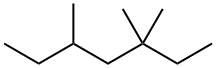# DAT Sample Questions & Answers

Here is a mini Dental Admissions Test (DAT) for quantitative reasoning and the survey of the natural sciences. To simulate a real Dental Admissions Test (DAT) you should give yourself no more than 19 minutes for answering all the questions on this test.

#### Biology

1. Exocytosis occurs when this organelle’s membrane fuses with the plasma membrane.
A.    Secretory vesicle
B.    Nucleus
C.    Mitochondrion
D.    Ribosome
E.    Golgi body
2. NAD and FAD are important molecules in living systems because they
A.    cause the synthesis of ATP from ADP and phosphate.
B.    serve as oxidation-reduction coenzymes.
C.    can reduce the activation energy of a biological reaction.
D.    control the level of acetyl Co-A in the cell.
E.    are essential reactants in those reactions called dehydration reactions.
3. The addition of potassium iodide as a nutritional supplement to common table salt would most directly affect the function of which of these glands?
A.    Thyroid
B.    Sweat glands
D.    Kidneys
E.    Parathyroid
4. The outer layer of cells, the ectoderm, in a developing embryo gives rise to the
A.    muscle system.
B.    reproductive system.
C.    circulatory system.
D.    skeletal system.
E.    nervous system.
5. Removal of the gallbladder makes it more difficult to digest foods high in
A.    carbohydrates.
B.    nucleic acids.
C.    proteins.
D.    fats.
E.    vitamins.

#### Inorganic Chemistry

6. Supercooled water at −10°C can spontaneously warm to 0°C in a perfectly insulated container. This is possible because
A.    supercooled materials do not obey energy conservation laws.
B.    supercooled water needs less energy for change of temperature than ordinary water.
C.    a small amount of energy is given to the surroundings.
D.    some of the water freezes and this provides energy.
E.    ice is unusual in that it is less dense than liquid water.
7. How many grams of NaOH (40.0 g/mol) are required to make 250 ml of a 0.500 M solution?
A.    (250)(0.500)
B.    (250)(500)/1000
C.    (250)(0.500)(40.0)/1000
D.    1000/(250)(0.500)(40.0)
E.    (250)(0.500)(40.0)
8. What is the percentage of oxygen by weight in Zn(H2PO4)2 (259g/mol)?
A.    53.3%
B.    24.7%
C.    39.5%
D.    6.18%
E.    49.4%
9. At 0°C and 1.0 atmosphere pressure (STP) 22.0 grams of gas occupies 11.2 liters. The gas could be which of the following?
A.    CO2 = 44g/mol
B.    CO = 28g/mol
C.    NH3 = 17g/mol
D.    CH4 = 16g/mol
E.    C4H10 = 58g/mol
10. When the following equation is properly balanced, the coefficient of H2O is Mg3N2 + H2O → Mg(OH)2 + NH3
A.    9
B.    6
C.    4
D.    3
E.    2

#### Organic Chemistry

11. Which of the following compounds would have the highest boiling point?
A.   CH3CH2CH2CH3
B.    CH3NH2
C.    CH3OH
D.    CH2F2
12. Which reaction below is a possible termination step in the free radical chlorination of CH3CH2CH2CH3 by Cl2?
A.    CH3CH2CH2CH3 + Cl → CH3CH2CHCH3 + HCl
B.    CL2 → Cl + Cl
C.    CH3CH2CH2CH2 + Cl → CH3CH2CH2CH2Cl
D.    CH3CH2CH2CH3 + Cl → CH3CH2CH2CH2 + HCl
E.    CH3CH2CHCH3 + CL2 → CH3CH2CHCH3 + Cl
13. Which of the following compounds is the strongest Brønsted base?
A.       CH4
B.        NH3
C.        H2O
D.        HF
14. Which of the following compounds is the strongest Brønsted base?
A.     H2PO4-
B.    HSO4-
C.    NO3-
D.     CH3COO-
15. The IUPAC name for the compound shown below is:A.    3,5-Dimethyl-3-ethylhexane
B.    4,4-Dimethyl-2-ethylhexane
C.    Trimethylheptane
D.    3,3,5-Trimethylheptane
E.    3,5-Trimethylheptane

#### PART 2 Quantitative Reasoning

16. 1/4 + 2/5 − 1/6 = ?
A.    −1/60
B.    2/15
C.    29/60
D.    8/15
E.    49/60
17. A person’s earnings increased by 10% from Year 1 to Year 2, and decreased 10% from Year 2 to Year 3. Which of the following percentages represents the change from Year 1 to Year 3?
A.    +20
B.    +1
C.    0
D.    −1
E.    −11
18. \$180 is to be shared by Bob and Frank so that Frank gets 25% more than Bob. How much does Bob get?
A.    \$72
B.    \$80
C.    \$100
D.    \$108
E.    \$144
19. A box of clarinet reeds sells for \$8 but is on sale at 25% off. If there is a sales tax of 6%, the total cost of one box is
A.    \$5.52
B.    \$5.65
C.    \$6
D.    \$6.36
E.    \$6.48
20. 42−1/6 = 0.1x What is x?
A.    7
B.    25.2
C.    70
D.    252
E.    2520

#### Part 1 Survey of Natural Science

1. A     6. D     11. C
2. B     7. C     12. C
3. A     8. E     13. B
4. E     9. A     14. D
5. D     10. B     15. D

#### Part 2 Quantitative Reasoning

16. C
17. D
18. B
19. D
20. E

You have to login to post comment!!